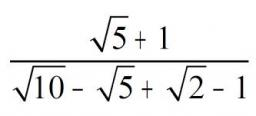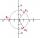# Calculation

How much is sum of square root of six and the square root of 225?

x =  17.45

### Step-by-step explanation:

$x=\sqrt{6}+\sqrt{225}=17.45$Did you find an error or inaccuracy? Feel free to write us. Thank you!## Related math problems and questions:

• Three members GPThe sum of three numbers in GP (geometric progression) is 21 and the sum of their squares is 189. Find the numbers.
• Exponential equationFind x, if 625 ^ x = 5 The equation is exponential because the unknown is in the exponential power of 625
• The sumThe sum of the first 10 members of the arithmetic sequence is 120. What will be the sum if the difference is reduced by 2?
• Annual incomeThe annual income (in thousands of \$) of fifteen families is 60, 80, 90, 96, 120, 150, 200, 360, 480, 520, 1060, 1200, 1450, 2500, 7200. Calculate the harmonic and geometric mean.
• Square root 2If the square root of 3m2 +22 and -x = 0, and x=7, what is m?
• The squareThe square root of 25 times the square root of 81 is what number?
• Sum of seriesDetermine the 6-th member and the sum of a geometric series: 5-4/1+16/5-64/25+256/125-1024/625+....
• Recursion squaresIn the square, ABCD has inscribed a square so that its vertices lie at the centers of the sides of the square ABCD. The procedure of inscribing the square is repeated this way. The side length of the square ABCD is a = 22 cm. Calculate: a) the sum of peri
• SquareSuppose the square's sides' length decreases by a 25% decrease in the content area of 28 cm2. Determine the side length of the original square.
• Geometric sequenceIn the geometric sequence is a4 = 20 a9 = -160. Calculate the first member a1 and quotient q.
• RemaindersIt is given a set of numbers { 170; 244; 299; 333; 351; 391; 423; 644 }. Divide this numbers by number 66 and determine set of remainders. As result write sum of this remainders.
• Digits A, B, CFor the various digits A, B, C is true: the square root of the BC is equal to the A and sum B+C is equal to A. Calculate A + 2B + 3C. (BC is a two-digit number, not a product).
• The projectileThe projectile was fired horizontally from a height of h = 25 meters above the ground at a speed of v0 = 250 m/s. Find the range and flight time of the projectile.
• Squares above sidesIn a right triangle, the areas of the squares above its sides are 169; 25 and 144. The length of its longer leg is:
• TriangleDetermine whether we can make a triangle with the given side lengths. If so, use Heron's formula to find the area of the triangle. a = 158 b = 185 c = 201
• Moivre 2Find the cube roots of 125(cos 288° + i sin 288°).
• Four prismsQuestion No. 1: The prism has the dimensions a = 2.5 cm, b = 100 mm, c = 12 cm. What is its volume? a) 3000 cm2 b) 300 cm2 c) 3000 cm3 d) 300 cm3 Question No.2: The prism base is a rhombus with a side length of 30 cm and a height of 27 cm. The height of t